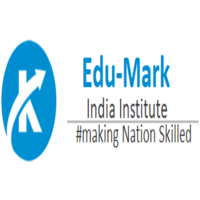﻿ Advanced Mathematics Preparation for JEE/CET/CAT - TutorialspointSubscription Includes

# What Will I Get ?

• Mathematical Logics with statements and Sentences
• Matrices and their Basic Operation and types
• Trigonometric Function and Problems regarding Trigonometry
• Problems related to pair of Straight Line
• Vector Operations , Using vector understand the logic and need of it
• Lines & Planes
• Linear Programming (LP) and how to study them and future use of it

# Requirements

• You should be comfortable with arithmetic (addition, subtraction, multiplication, division) of whole numbers.
• You should be comfortable with arithmetic of decimals, fractions, exponents, and radicals.
• We'll start the course with basic understanding and knowledge of algebraic operations, so if you know those arithmetic basics, you'll be well prepared for the course.

# Mathematics is considered as one of the challenging subjects, therefore, we have designed this course by keeping in mind all the needs of students.

### :::: The chapters covered are (Advanced Math GRADE 11-12):::::

1.  Mathematical Logic
2. Matrics
3. Trigonometric function
4. Pair of Straight line
5. Linear Programming
6. Line and Plane

### :::: Who are your target students? :::::

• Mathematician facing problems in Algebra and Advance mathematics likes ALGEBRA & GEOMETRY
• Beginner Class for Mathematics and Advance ALGEBRA & GEOMETRY
• A beginner who is curious for mathematics like Linear Programming, Logic, Matrices, Trigonometry, Geometry Theorems
• Homeschool parents looking for extra support with Mathematic
• Anyone who wants to study advanced mathematics, ALGEBRA & GEOMETRYfor fun after being away from school for a while

### ::: What will you Learn in THIS COURSE:::

• Mathematical Logics with statements and Sentences
• Matrices and their Basic Operation and types
• Trigonometric Function and Problems regarding Trigonometry
• Problems related to pair of Straight Line
• Lines & Plane
• Linear Programming (LP) and how to study them and future use of it

### :::: What ARE the benefits for this COURSE:::::

• On completion of this course, one will have detailed knowledge of all the chapters and can easily solve all the problems which can lead to score well in exams with the help of:
• Explanatory videos ensure complete concept understanding.

• Quiz Video helps in testing your knowledge

• In short, it is an interesting course fulfilling all the student's needs.

• Certificate after Accomplishing the course

• QUARTERLY(3months) UPDATES ON THIS COURSE

# Course Content